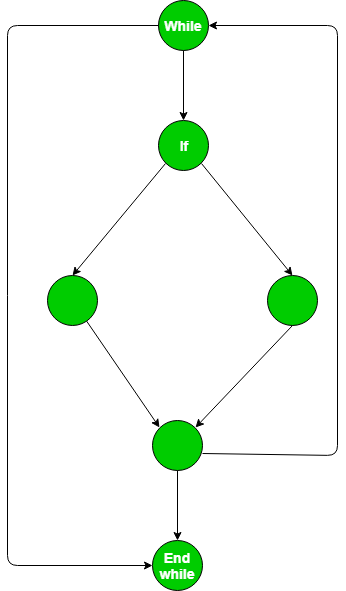GeeksforGeeks App
Open AppBrowser
Continue

# ISRO | ISRO CS 2014 | Question 4

Consider the following pseudo code

```while (m < n)
if (x > y ) and (a < b) then
a=a+1
y=y-1
end if
m=m+1
end while ```

What is cyclomatic complexity of the above pseudo code?
(A) 2
(B) 3
(C) 4
(D) 5

Explanation: Draw the Control Flow Graph (CFG) for given pseudo code:Now, cyclomatic complexity:

```= (number of edges) - (number of nodes) + 1 = 8-6+2 = 4
= Total number of regions = 4
= Total number of decision points + 1 = 3+1 = 4 ```

Note that decision points at a node = (total number of outgoing edges) – 1.

So, option (C) is correct.

Quiz of this Question

My Personal Notes arrow_drop_up